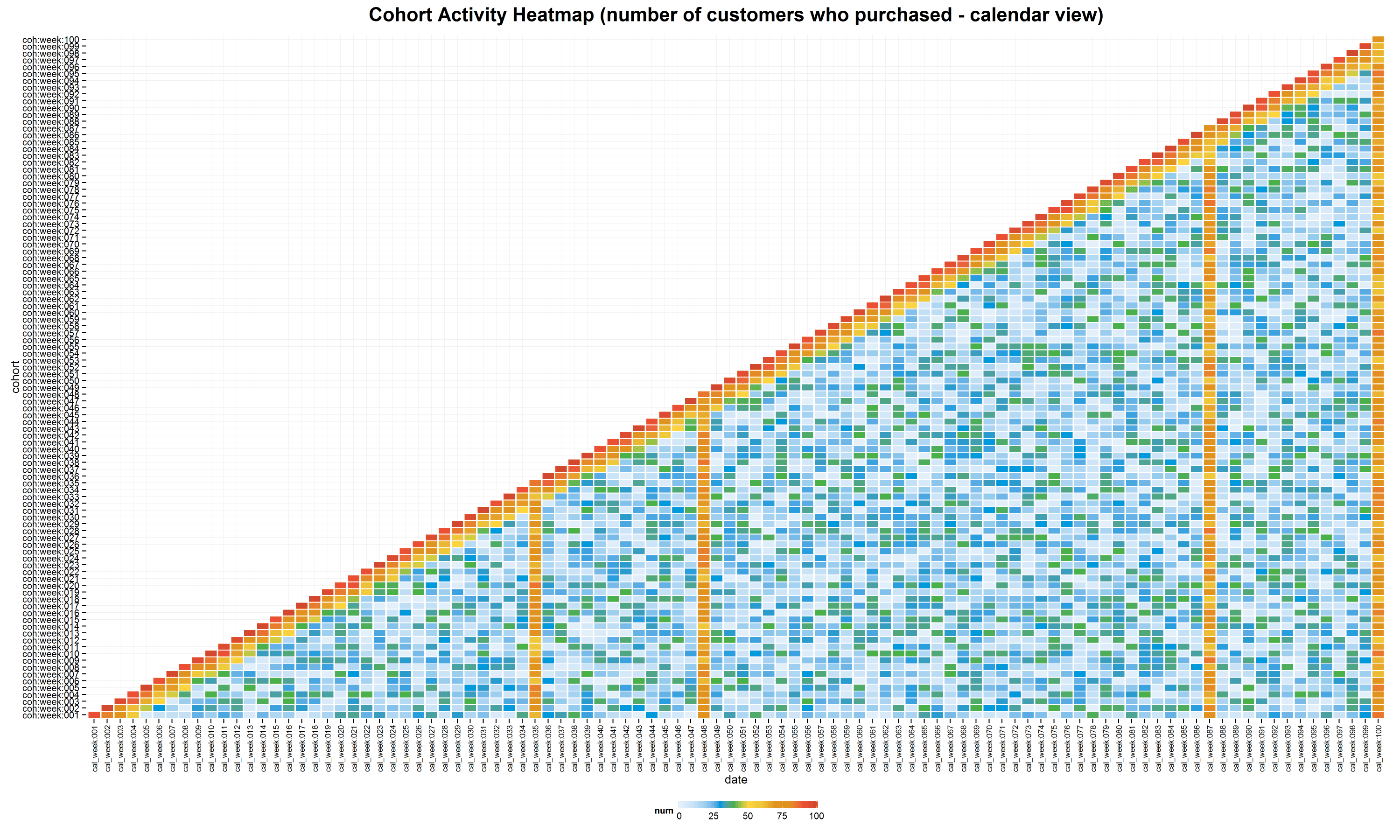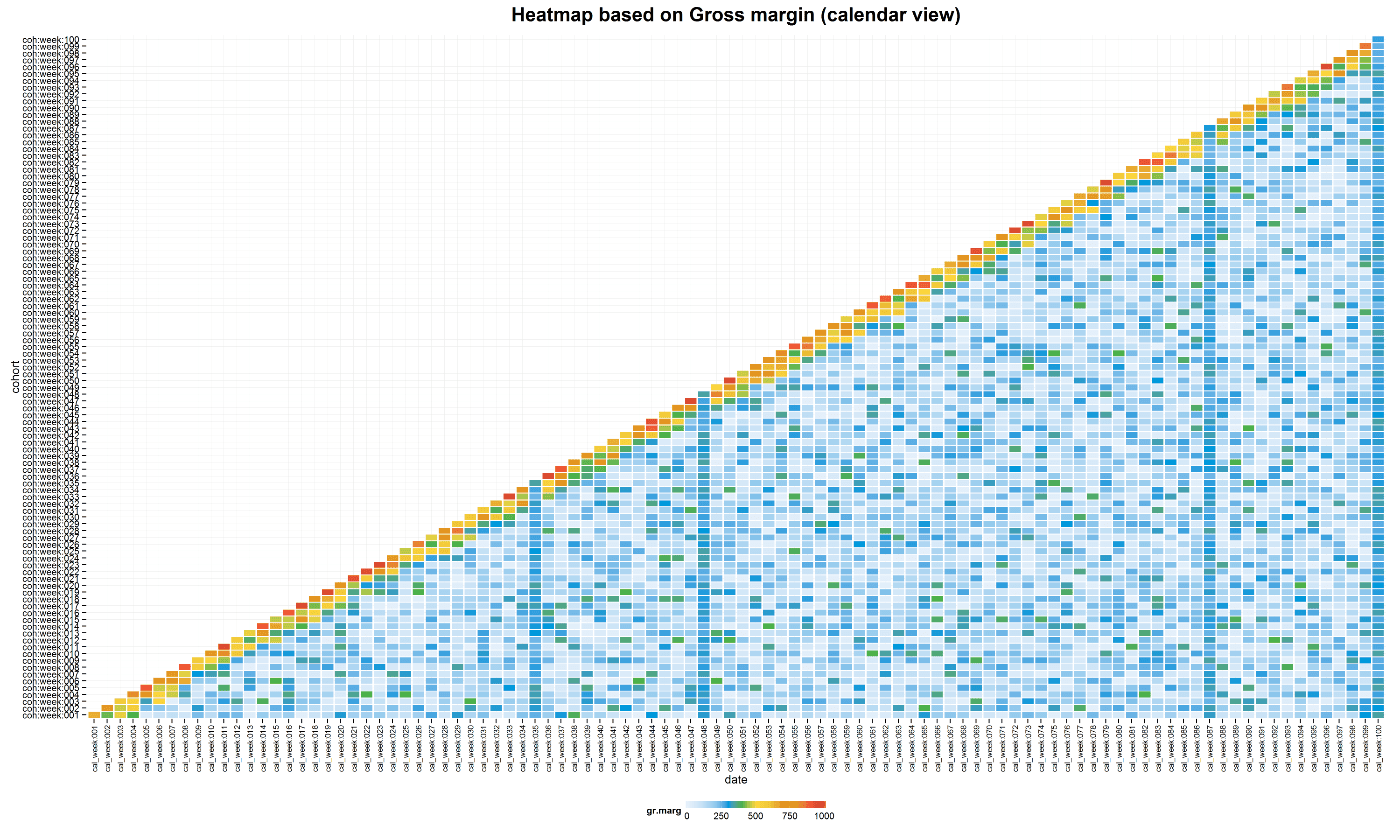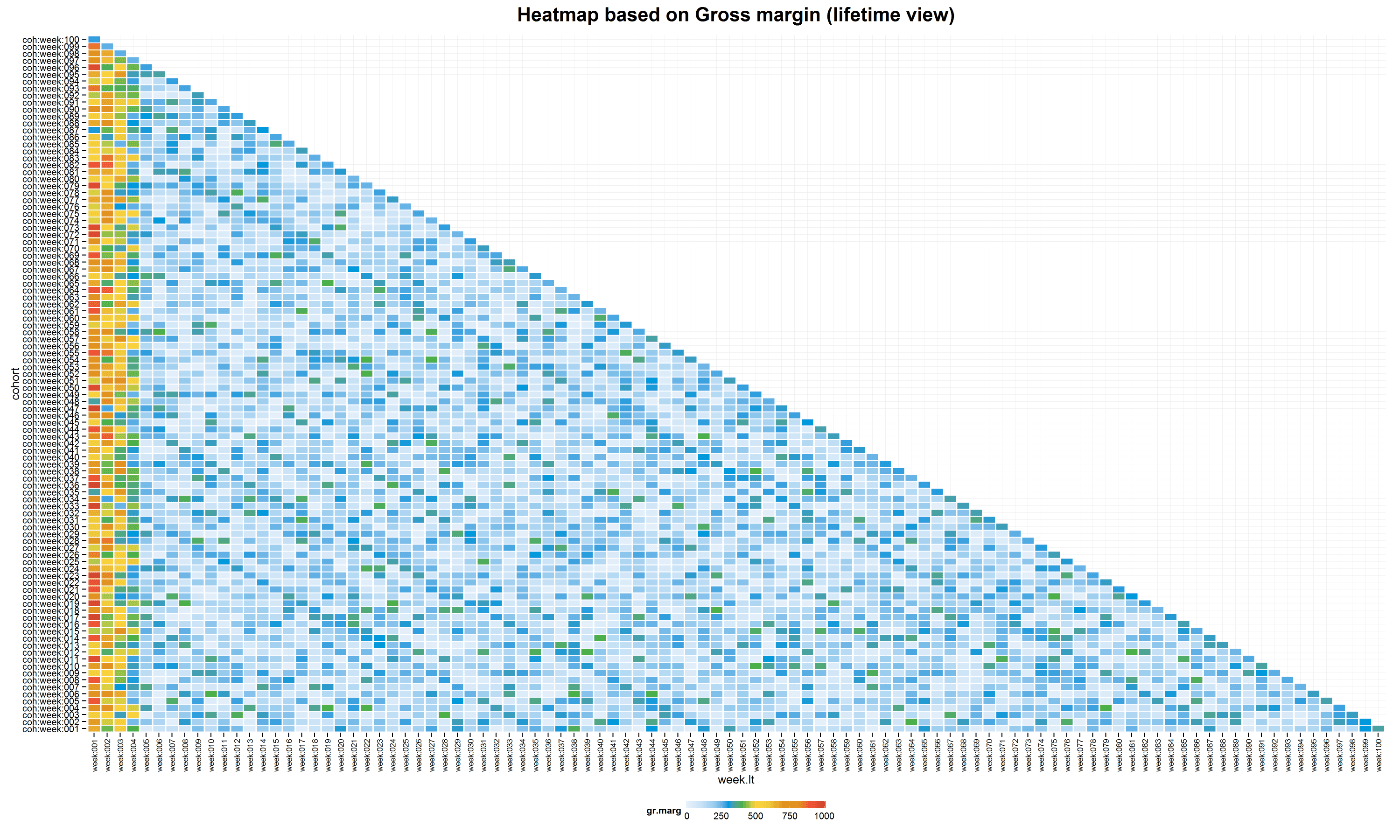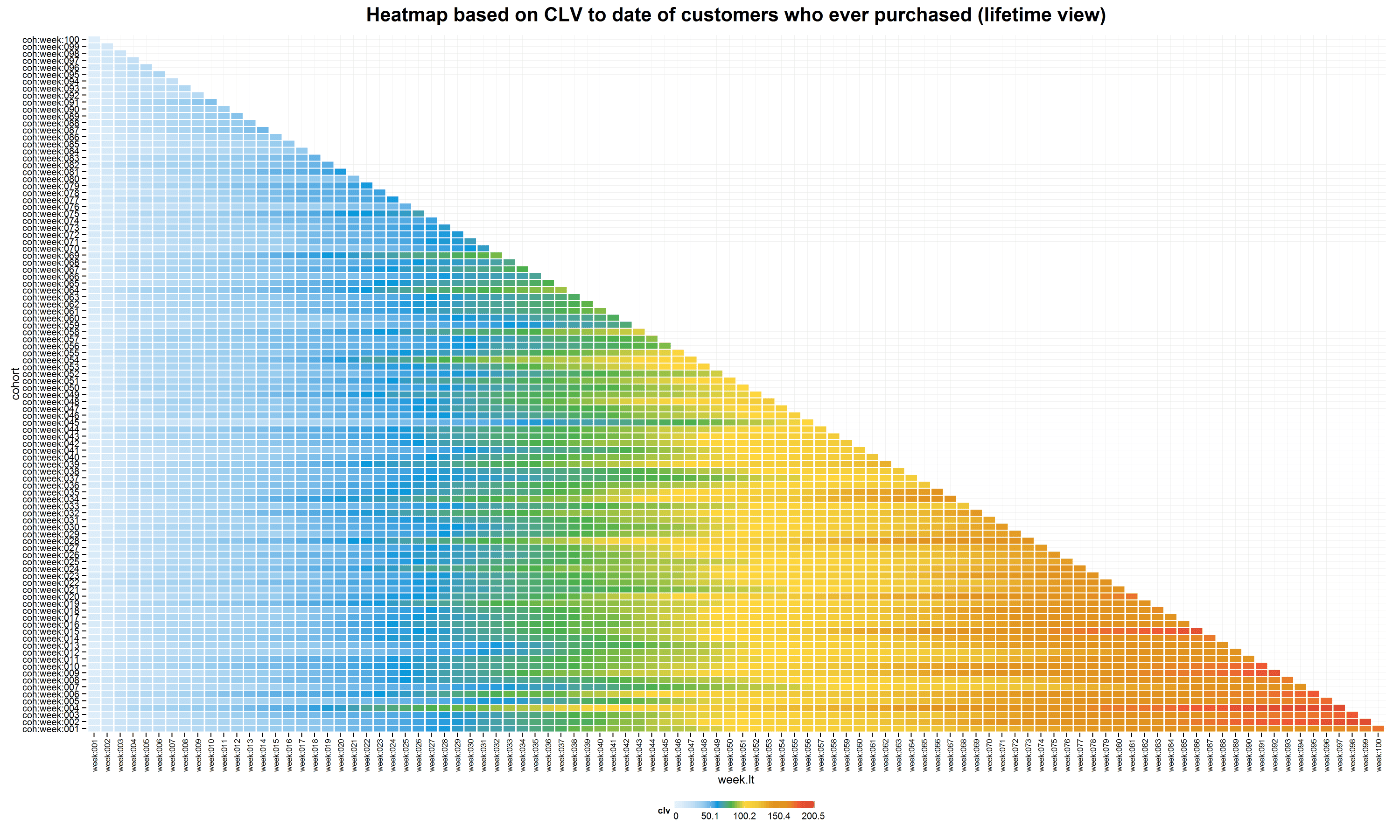Cohort Analysis with Heatmap

Previously I shared the data visualization approach for the descriptive analysis of progress of cohorts with the “layer-cake” chart (part I and part II). In this post, I want to share another interesting visualization that not only can be used for descriptive analysis as well but would be more helpful for analyzing a large number of cohorts.

For instance, if you need to form and analyze weekly cohorts, you would have 52 cohorts within a year.

The Heatmap chart would be helpful for primary analysis and we will study how to create it with the R programming language. But firstly, I would like to give credit to John Egan who shared the idea of using the Cohort Activity Heatmap and to Ben Moore whose great post helped me to reproduce such a beautiful color palette.

The following is my interpretation of using the Heatmap for Cohort Analysis.

Let’s assume we form weekly cohorts and have 100 ones as of the reporting date. We’ve tracked the number of customers who made a purchase and the total gross margin per weekly cohort per time lapse (a week in our case). We can easily calculate two extra values based on these data:

• per customer gross margin per cohort per week,
• customer lifetime value (CLV) to date as accumulated gross margin per cohort divided by an initial number of customers in the cohort.

In addition, I’ve simulated some purchase patterns that can be plausible, specifically:

• customers tend to buy actively during the first weeks of the lifetime,
• we have two seasonal growths of sales every year (e.g. Back to school sales and Black Friday that are accompanied with higher discounts).

Based on these data we can plot at least four types of charts using Heatmap:

1. Cohort activity, based on the number of customers who made a purchase each week (active customers),
2. Cohort gross margin, based on the total amount of money that the cohort brought each week,
3. Per customer gross margin, based on the average gross margin that the cohort brought each week,
4. Cohort CLV to date, based on cumulative CLV to date.

Furthermore, charts can be represented based on calendar dates and the serial number of the week of the lifetime (e.g. 1st week, 2nd week, etc. from the first purchase date) as well. Therefore, we can see the influence of seasonality or other occurrences on all existing cohorts as of calendar date and the progress of each cohort comparing to the others based on the serial number of the week of the lifetime.

And our eight charts are the following:We have placed dates (calendar or week of lifetime) on the x-axis and cohorts on the y-axis. The color of the heatmap represents the value (number of customers, gross margin, per customer gross margin and CLV to date).

Based on this type of visualization we can easily identify general purchasing behaviors, for instance:

• number of customers who made a purchase was at its highest at the beginning of the cohort’s lifetime (first four weeks) and has increased in the sales seasons,
• although the number of customers who made purchases increased in the sales seasons, the gross margin hasn’t increased accordingly. In other words, lower prices haven’t  been compensated by a higher number of customers,
• if our average customer acquisition cost (CAC) is \$50, for example, we can find that almost all cohorts have been repaid by CLV to date within the 32-35 weeks of lifetime,
• and so on.

You can produce this example via the following R code:

click to expand R code

```#loading libraries
library(dplyr)
library(ggplot2)
library(reshape2)

#simulating dataset
cohorts <- data.frame()
set.seed(10)
for (i in c(1:100)) {
coh <- data.frame(cohort=i,
date=c(i:100),
week.lt=c(1:(100-i+1)),
num=replicate(1, sample(c(1:40), 100-i+1, rep=TRUE)),
av=replicate(1, sample(c(5:10), 100-i+1, rep=TRUE)))
coh\$num[coh\$week.lt==1] <- sample(c(90:100), 1, rep=TRUE)
ifelse(max(coh\$date)>1, coh\$num[coh\$week.lt==2] <- sample(c(75:90), 1, rep=TRUE), NA)
ifelse(max(coh\$date)>2, coh\$num[coh\$week.lt==3] <- sample(c(60:75), 1, rep=TRUE), NA)
ifelse(max(coh\$date)>3, coh\$num[coh\$week.lt==4] <- sample(c(40:60), 1, rep=TRUE), NA)
ifelse(max(coh\$date)>34, {
coh\$num[coh\$date==35] <- sample(c(60:85), 1, rep=TRUE)
coh\$av[coh\$date==35] <- 4
}, NA)
ifelse(max(coh\$date)>47, {
coh\$num[coh\$date==48] <- sample(c(60:85), 1, rep=TRUE)
coh\$av[coh\$date==48] <- 4
}, NA)
ifelse(max(coh\$date)>86, {
coh\$num[coh\$date==87] <- sample(c(60:85), 1, rep=TRUE)
coh\$av[coh\$date==87] <- 4
}, NA)
ifelse(max(coh\$date)>99, {
coh\$num[coh\$date==100] <- sample(c(60:85), 1, rep=TRUE)
coh\$av[coh\$date==100] <- 4
}, NA)

coh\$gr.marg <- coh\$av*coh\$num
cohorts <- rbind(cohorts, coh)
}

cohorts\$cohort <- formatC(cohorts\$cohort, width=3, format='d', flag='0')
cohorts\$cohort <- paste('coh:week:', cohorts\$cohort, sep='')
cohorts\$date <- formatC(cohorts\$date, width=3, format='d', flag='0')
cohorts\$date <- paste('cal_week:', cohorts\$date, sep='')
cohorts\$week.lt <- formatC(cohorts\$week.lt, width=3, format='d', flag='0')
cohorts\$week.lt <- paste('week:', cohorts\$week.lt, sep='')

#calculating CLV to date
cohorts <- cohorts %>%
group_by(cohort) %>%
mutate(clv=cumsum(gr.marg)/num[week.lt=='week:001']) %>%
ungroup()

#color palette
cols <- c("#e7f0fa", "#c9e2f6", "#95cbee", "#0099dc", "#4ab04a", "#ffd73e", "#eec73a", "#e29421", "#e29421", "#f05336", "#ce472e")

#Heatmap based on Number of active customers
t <- max(cohorts\$num)

ggplot(cohorts, aes(y=cohort, x=date, fill=num)) +
theme_minimal() +
geom_tile(colour="white", width=.9, height=.9) +
breaks=seq(0, t, by=t/4),
labels=c("0", round(t/4*1, 1), round(t/4*2, 1), round(t/4*3, 1), round(t/4*4, 1)),
guide=guide_colourbar(ticks=T, nbin=50, barheight=.5, label=T, barwidth=10)) +
theme(legend.position='bottom',
legend.direction="horizontal",
plot.title = element_text(size=20, face="bold", vjust=2),
axis.text.x=element_text(size=8, angle=90, hjust=.5, vjust=.5, face="plain")) +
ggtitle("Cohort Activity Heatmap (number of customers who purchased - calendar view)")

ggplot(cohorts, aes(y=cohort, x=week.lt, fill=num)) +
theme_minimal() +
geom_tile(colour="white", width=.9, height=.9) +
breaks=seq(0, t, by=t/4),
labels=c("0", round(t/4*1, 1), round(t/4*2, 1), round(t/4*3, 1), round(t/4*4, 1)),
guide=guide_colourbar(ticks=T, nbin=50, barheight=.5, label=T, barwidth=10)) +
theme(legend.position='bottom',
legend.direction="horizontal",
plot.title = element_text(size=20, face="bold", vjust=2),
axis.text.x=element_text(size=8, angle=90, hjust=.5, vjust=.5, face="plain")) +
ggtitle("Cohort Activity Heatmap (number of customers who purchased - lifetime view)")

# Heatmap based on Gross margin
t <- max(cohorts\$gr.marg)

ggplot(cohorts, aes(y=cohort, x=date, fill=gr.marg)) +
theme_minimal() +
geom_tile(colour="white", width=.9, height=.9) +
breaks=seq(0, t, by=t/4),
labels=c("0", round(t/4*1, 1), round(t/4*2, 1), round(t/4*3, 1), round(t/4*4, 1)),
guide=guide_colourbar(ticks=T, nbin=50, barheight=.5, label=T, barwidth=10)) +
theme(legend.position='bottom',
legend.direction="horizontal",
plot.title = element_text(size=20, face="bold", vjust=2),
axis.text.x=element_text(size=8, angle=90, hjust=.5, vjust=.5, face="plain")) +
ggtitle("Heatmap based on Gross margin (calendar view)")

ggplot(cohorts, aes(y=cohort, x=week.lt, fill=gr.marg)) +
theme_minimal() +
geom_tile(colour="white", width=.9, height=.9) +
breaks=seq(0, t, by=t/4),
labels=c("0", round(t/4*1, 1), round(t/4*2, 1), round(t/4*3, 1), round(t/4*4, 1)),
guide=guide_colourbar(ticks=T, nbin=50, barheight=.5, label=T, barwidth=10)) +
theme(legend.position='bottom',
legend.direction="horizontal",
plot.title = element_text(size=20, face="bold", vjust=2),
axis.text.x=element_text(size=8, angle=90, hjust=.5, vjust=.5, face="plain")) +
ggtitle("Heatmap based on Gross margin (lifetime view)")

# Heatmap of per customer gross margin
t <- max(cohorts\$av)

ggplot(cohorts, aes(y=cohort, x=date, fill=av)) +
theme_minimal() +
geom_tile(colour="white", width=.9, height=.9) +
breaks=seq(0, t, by=t/4),
labels=c("0", round(t/4*1, 1), round(t/4*2, 1), round(t/4*3, 1), round(t/4*4, 1)),
guide=guide_colourbar(ticks=T, nbin=50, barheight=.5, label=T, barwidth=10)) +
theme(legend.position='bottom',
legend.direction="horizontal",
plot.title = element_text(size=20, face="bold", vjust=2),
axis.text.x=element_text(size=8, angle=90, hjust=.5, vjust=.5, face="plain")) +
ggtitle("Heatmap based on per customer gross margin (calendar view)")

ggplot(cohorts, aes(y=cohort, x=week.lt, fill=av)) +
theme_minimal() +
geom_tile(colour="white", width=.9, height=.9) +
breaks=seq(0, t, by=t/4),
labels=c("0", round(t/4*1, 1), round(t/4*2, 1), round(t/4*3, 1), round(t/4*4, 1)),
guide=guide_colourbar(ticks=T, nbin=50, barheight=.5, label=T, barwidth=10)) +
theme(legend.position='bottom',
legend.direction="horizontal",
plot.title = element_text(size=20, face="bold", vjust=2),
axis.text.x=element_text(size=8, angle=90, hjust=.5, vjust=.5, face="plain")) +
ggtitle("Heatmap based on per customer gross margin (lifetime view)")

# Heatmap of CLV to date
t <- max(cohorts\$clv)

ggplot(cohorts, aes(y=cohort, x=date, fill=clv)) +
theme_minimal() +
geom_tile(colour="white", width=.9, height=.9) +
breaks=seq(0, t, by=t/4),
labels=c("0", round(t/4*1, 1), round(t/4*2, 1), round(t/4*3, 1), round(t/4*4, 1)),
guide=guide_colourbar(ticks=T, nbin=50, barheight=.5, label=T, barwidth=10)) +
theme(legend.position='bottom',
legend.direction="horizontal",
plot.title = element_text(size=20, face="bold", vjust=2),
axis.text.x=element_text(size=8, angle=90, hjust=.5, vjust=.5, face="plain")) +
ggtitle("Heatmap based on CLV to date of customers who ever purchased (calendar view)")

ggplot(cohorts, aes(y=cohort, x=week.lt, fill=clv)) +
theme_minimal() +
geom_tile(colour="white", width=.9, height=.9) +
breaks=seq(0, t, by=t/4),
labels=c("0", round(t/4*1, 1), round(t/4*2, 1), round(t/4*3, 1), round(t/4*4, 1)),
guide=guide_colourbar(ticks=T, nbin=50, barheight=.5, label=T, barwidth=10)) +
theme(legend.position='bottom',
legend.direction="horizontal",
plot.title = element_text(size=20, face="bold", vjust=2),
axis.text.x=element_text(size=8, angle=90, hjust=.5, vjust=.5, face="plain")) +
ggtitle("Heatmap based on CLV to date of customers who ever purchased (lifetime view)")

```